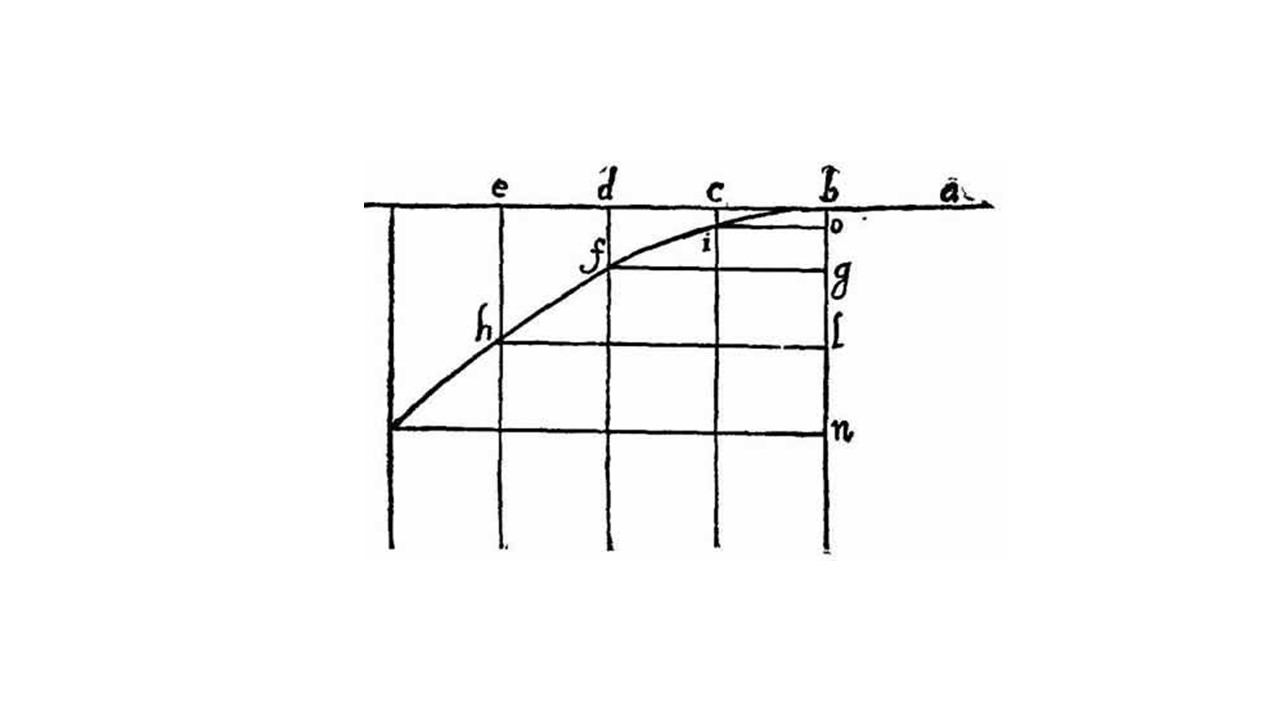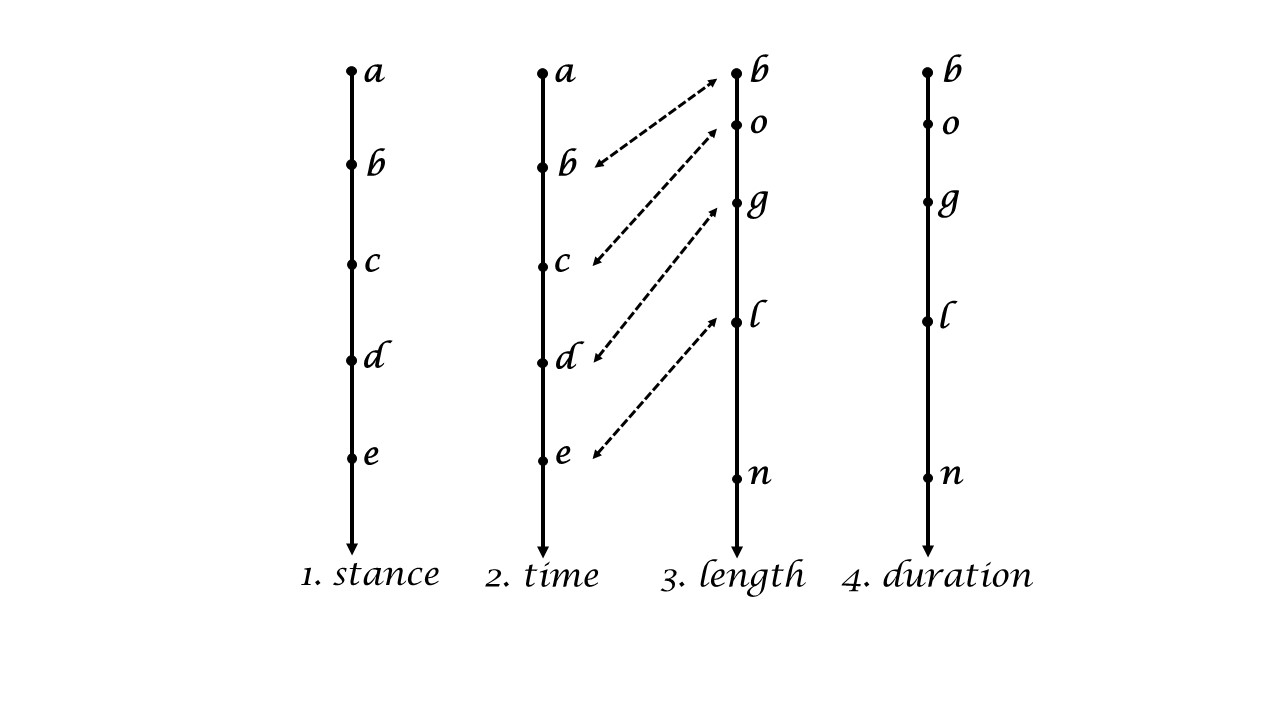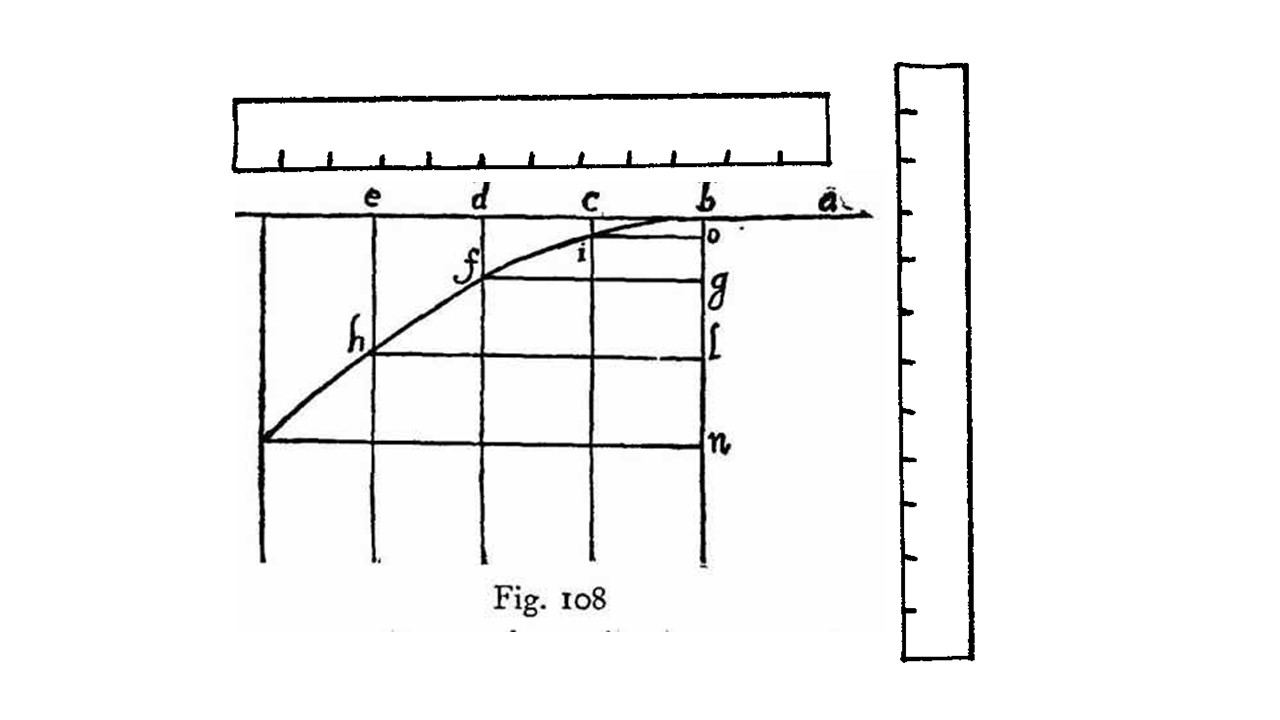iSoul In the beginning is reality.

# Independent variable dimension

This continues the series of posts, see here.

Let’s begin with Galileo’s figure for uniform motion and uniform accelerated motion:Let the horizontal uniform motion be situated in a 2D x, y coordinate system:The dependent uniform acceleration moves in an additional dimension, z, and so has 3D coordinates.

If the independent uniform motion is measured by time, then time has 2D coordinates. The coordinates are proportional to one another, and so may just as well be replaced by a scalar of the signed magnitude. The same can be done if the uniform motion has 3D coordinates. The scalar time is proportional to the common measure of time, with the appropriate rate of motion, it is the same as scalar time.

One can say the same if the independent uniform motion is measured by length, then length has 2D (or 3D) coordinates. This is nothing new, but because of the uniform motion, the coordinate lengths are proportional to one another, and so may just as well be replaced by a single scalar of the signed magnitude. In this way, length becomes a scalar, called here the stance.

# Motion ordered by time or stance

This continues posts about Galileo such as here. Look again at this figure from Galileo:The horizontal line represents the independent variable, which is the horizontal component of a projectile with an initial speed that falls with uniform acceleration. The independent variable need not be horizontal, so let us represent it vertically with the vertical component of motion:The first two lines represent the independent uniform motion but are measured differently, (1) by stance and (2) by time. The second and third lines show the correspondence between the independent variable (time) and the dependent variable (length). The first and fourth lines have the same relation: stance is the independent variable and duration is the dependent variable. Lines 1 and 2 are proportional, as are lines 3 and 4. As the units change, the functional relationship does not: the dependent variable is proportional to a square of the independent variable.

Time is the measure of duration of a uniform motion that is the reference motion, which means it is the independent variable. Similarly, stance is the measure of length of a uniform motion that is the reference motion, in which case it is the independent variable. The dependent variable for stance is duration in three dimensions, whereas for time the dependent variable is length in three dimensions.

# Timeframes of reference

A kinematic frame of reference is a mathematical method to determine the position of points in abstract 3D space and scalar time. An inertial frame of reference is a physical method to measure the position of bodies in physical 3D space and scalar time. The latter is often envisioned as three mutually-perpendicular rigid rods attached at a common point, or a lattice of such rigid rods. In addition, there is envisioned a clock at every node of the lattice, which are all synchronized, which requires a method to synchronize them. The common point is called the origin point.

Such a frame of reference assigns coordinates in 3D space and 1D time to every event.A kinematic timeframe of reference is a mathematical method to determine the position of points in abstract scalar space and 3D time. An inertial timeframe of reference is a physical method to measure the position of bodies in physical 3D space and scalar time. The latter may be envisioned as three mutually-perpendicular rigid monorails attached at a common point, or a lattice of such rigid monorails, with a regular succession of small monorail vehicles traveling at constant velocity. In addition, there is envisioned a metreloge at every node of the lattice, which are all synstancized, which requires a method to synstancize them. The start of monorails leaving the common point is the origin event.

Such a timeframe of reference assigns coordinates in 1D space and 3D time to every event.# Places, spaces, and times

Time is like a river that flows on indefinitely, as observed from a place on its bank. The flow of time is downstream. Place does not change in this way but the time keeps changing.

Space is like a river that flows on indefinitely, as observed from a platform floating down the river. The flow of space is upstream, as places on the bank recede from view. Time does not change in this way but the place keeps changing.

Places have spaces between them. Spaces are distances measured as lengths (length of space). Places are also called stations, as in railroad stations, if they are places along a route (stance and station are related etymologically). Spaces are located by the places at their beginning and end points. “What station is it here?” could be asked by a passenger in a train at a stop.

Times have time intervals between them. Time intervals are distances measured as durations (length of time). Times are chronated (positioned) in 3D time. Time intervals are chronated by the times at their beginning and end instants. “What time is it now?” could be asked in many contexts.

Spacetime is a place-based metric. Timespace is a time-based metric.

In classical physics there is a conversion factor between space and time that is adopted as a convention by all observers and is measured by a uniform motion relative to each observer. In relativity physics there is a uniform motion that is absolute, that is, the same as measured by every observer, and functions as a conversion factor between space and time.

# From spacetime to space and time

This relates to the post here.

There are three dimensions of motion with two measures of the extent of motion, which makes a total of six metric dimensions of motion. But these six metric dimensions collapse into two structures of one and three dimensions as the conversion factor approaches infinity.

The invariant proper length, , is:

dσ² = dr²dt²/ç² = dr1² + dr2² + dr3² – dt²/ç² = dr² – dt1²/ç² – dt2²/ç² – dt3²/ç² = dr1² + dr2² + dr3² – dt1²/ç² – dt2²/ç² – dt3²/ç².

As the conversion factor, ç, the pace of light, approaches infinity, this becomes

dσ² = dr² = dr1² + dr2² + dr3².

That is, the time coordinates separate from the invariant length, which becomes the Euclidean distance of three dimensional space. Time is left as an invariant scalar called the time.

The invariant proper time, , is:

dτ² = dσ²/c² = dr²/c² – dt² = (dr1² + dr2² + dr3²)/c² – dt² = dr²/c² – dt1² – dt2² – dt3² = (dr1² + dr2² + dr3²)/c² – dt1² – dt2² – dt3².

As the conversion factor, c, the speed of light, approaches infinity, this becomes

dτ² = – dt² = – dt² = – dt1² – dt2² – dt3².

That is, the length coordinates separate from the invariant time, which becomes the Euclidean distime of three dimensional time. Space is left as an invariant scalar called the stance.

The result is that six dimensional spacetime collapses into 3D space with scalar time or 3D time with scalar space.

# Three kinds of empirical science

This post is related to an old post here.

Broadly speaking, there are three kinds of empirical science, which correspond to three views of nature.

(1) The ancient view of empirical science is represented by Aristotle, which includes the careful observation of undisturbed nature. Motion, for example, meant natural motion, not “violent” motion in which there is a change of the natural course of things. Experimentation was not considered a way to understand undisturbed nature.

(2) The early modern view of empirical science includes experimentation because nature is understood to include what happens after an intervention in the course of nature. These experiments allowed early modern scientists to isolate causal factors in nature. The human observer was not considered part of any experiment.

(3) The late modern view of empirical science includes the observer as part of nature. The distinction between natural and artificial is discarded. The origin and nature of humans is included in his view of nature. Empirical science covers all aspects of human beings that can be observed. The scientist has a double life in which they both are and are not the object of science.

The second kind of empirical science is superior because it goes beyond the undisturbed nature of the first kind and does not include the contradiction at the heart of the third kind.

# Terminology contexts

This post continues the one here. While I avoid coining new terms or new definitions, some have been necessary. To have a consistent vocabulary, I try to imagine contexts in which they easily fit.

Some words are simply variations of words in use: distime is like distance; dischronment is like displacement; chronation is like location; vass is like mass; levitation is the opposite of gravitation; and oldtons are the units for rush, analogous to newtons for force. Metreloge is like horologe, which is a clock.

One context is racing. The term pace is used, particularly in running and (bi)cycling to mean the time interval per unit distance, which is the inverse of speed. The direction is ignored or assumed to follow the course of the race so a new term is needed to indicate the vector version of pace. For this I have chosen legerity, which is an old literary term for lightness of movement.

The second context is transport, such as package delivery. Consider an order to expedite a delivery. That means to reduce the time of transport, analogous to acceleration. A package stamped with “RUSH” gets a greater effort to reduce the time of delivery. Rush is analogous to a force applied. To hustle means to apply a rush over a distance, analogous to a force applied over time (which is called impulse). Surge is a rush applied over a dischronment, which is the inverse of work. Reserve is the capacity for surge, which is analogous to energy.

# Ratios of length and duration

This post relates to others such as this.

Consider Galileo’s figure (see his Dialogues Concerning Two New Sciences, tr. Crew & De Salvio p.249 Fig. 108 or Drake’s translation p.221) below with horizontal and vertical rulers added :A projectile moves with uniform velocity horizontally to the left and begins to descend at point b. Galileo used the sequence a-b-c-d-e to represent time and the sequence b-o-g-l-n to represent the height of the projectile above the Earth. The sequence b-i-f-h represents the parabolic path of the falling projectile.

Any uniform motion can serve as a reference motion. There are two uses of a reference variable: (i) as a parametric variable, or (ii) as a measurement variable. A parametric variable is an independent variable that provides ordered input for any dependent variable. A measurement variable is a variable that is dependent on the independent variable being measured. In the figure above the parametric variable is the time (duration) of the uniform motion on the horizontal axis, and the measurement variable is the height (length) of the uniform acceleration on the vertical axis.

Combine this with the two measures of motion, length and duration, and there are four possible cases: (1) independent duration variable with dependent length variable; (2) independent length variable with dependent duration variable; (3) independent length variable with dependent length variable; and (4) independent duration variable with dependent duration variable.

The figure above is an example of case (1). Its complement is case (2). Cases (3) and (4) include only one measure, length or duration, and so cannot express a rate of motion. Galileo expresses case (1) as a proportion between ratios of the variables at different times: s1 : s2 :: t12 : t22, which avoids combining different units in a single ratio, consistent with Eudoxian proportionality.

Consider case (2) in which the independent variable is length. This variable is a stanceline for locating other motions, which is like a timeline except that it expresses an independent length as the order parameter. The dependent reference variable in this case is duration, which measures any independent variable, in this case projectile height. This could be expressed as a proportion between ratios of the variables at different times: t1 : t2 :: s12 : s22, avoiding different units in a single ratio.

Case (1) enables multiple length variables dependent on one independent variable, the timeline. Case (2) enables multiple duration variables dependent on one independent variable, the stanceline. Rates of motion in case (1) are in units of the independent timeline, which is duration. Rates of motion in case (2) are in units of the independent stanceline, which is length.

# From length to duration and back

Let’s start with one-dimensional, i.e., scalar, functions, f, g, h, and k. Say there is the following functional relation:

s = f(t) = f(h(s)) ≡ g(s) = t,

t = g(s) = g(k(t)) ≡ f(t) = s,

in which s and t are parameters with different units. By implication the functions are either f or its inverse:

s = f(t) = f(f-1(s)) = t,

t = f-1(s) = f-1(f(t)) = s.

Function f takes t-units into s-units, and function f-1 takes s-units into t-units. The vector versions are as follows:

s = f(t) = f(f-1(s)) = t,

t = f-1(s) = f-1(f(t)) = s.

Motion space is an ordered pair of vectors s and t: (s, t), resulting in their direct sum vector space. Addition is conducted by components: (r, w) + (s, t) = (r + s, w + t). Scalar multiplication is also by component: (a, b) (s, t) = (as, bt). To multiply a scalar and only one component requires the other component to be unity. Thus additive unity is (0, 0) and multiplicative unity is (1, 1).

There are two ways to mask an ordered pair of vectors: left mask (s, t) = (s, t) and right mask (s, t) = (s, t), where s = |s| and t = |t|. What was described here as expansion and contraction may now be shown more clearly as masking and unmasking. A parametric length vector function is converted to a parametric duration vector function as follows:

r(t) = [r(t), θ(t), φ(t)] ↑ [(t´, χ´, ψ´), θ´(t´, χ´, ψ´), φ´(t´, χ´, ψ´)] ↔ ((r´, θ´, φ´), χ´(r´, θ´, φ´), ψ´(r´, θ´, φ´)) ↓ [t(r), χ(r), ψ(r)] = t(r).

# Intentional and extensional causes

This post continues previous posts on causes, especially the one here.

Final and formal causes constitute top-down causality, which may lead to efficient and material causes. Material and efficient (mechanism) causes constitute bottom-up causality, which may lead to formal and final causes. Top-down is intentional. Bottom-up is extensional.

The Inverse Causality Principle states that top-down causality is inverse of bottom-up causality.

The Inverse Correspondence Principle states that intentional motion is the inverse of extensional motion and experimentation is the inverse of observation. Similarly, transmission is the inverse of reception, developmental is the inverse of empirical, and time is the inverse of space.

The goal of science is empirical theory. The goal of engineering is development of something practical.

Goal and action go together like form and content or matter.

Consider Galileo dropping two balls, one wooden and one metal, from the tower of Pisa. One observer says it’s a race to the ground. Another observer says it’s an experiment. What is the nature of the balls? Or what does Nature do?

Final and formal causes are the inverse of efficient and material causes.